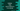# Python program to find out the sum of all numbers in an array## Find out the sum of all numbers in an array in Python:

In this post, we will learn how to find the sum of all numbers in an array using Python. We will see two different ways to solve it. First way is to looping through the array and find the sum by adding all values of the array and the other way is to find the sum directly by calling sum() method.

For example, if the array is [1,2,4,5,7], it will print 19.

### Steps to solve this problem:

We will use the below steps to solve it:

• Initialize one variable as 0 to hold the sum.
• Run one for loop and iterate through the elements of the array one by one.
• Add all elements to the sum variable.
• Print out the sum

### Method 1: Python program using a loop:

Below is the complete program:

``````given_array = [1,2,3,4,5]

sum = 0

for i in range(len(given_array)):
sum = sum + given_array[i]

print("Sum : ",sum)``````

It will print 15.

We can also create one different method to find the sum:

``````def findSum(arr):
sum = 0
for i in range(len(arr)):
sum = sum + arr[i]
return sum

given_array = [1,2,3,4,5]
print("Sum : ",findSum(given_array))``````

It prints the same output.

### Method 2: Python program using sum():

We can also use sum() method to find the sum of digits of an array directly:

``````given_array = [1,2,3,4,5]

print("Sum : ",sum(given_array))``````

It prints the same output.